# 5. a) Determine the molar solubility of PbSO4 in pure water, Ksp (PbSO4) = 1.82 *...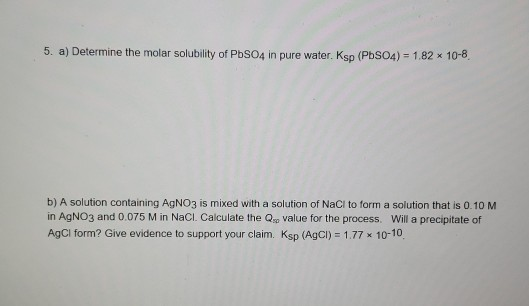5. a) Determine the molar solubility of PbSO4 in pure water, Ksp (PbSO4) = 1.82 * 10-8 b) A solution containing AgNO3 is mixed with a solution of NaCl to form a solution that is 0.10 M in AgNO3 and 0.075 M in NaCl. Calculate the value for the process. Will a precipitate of AgCl form? Give evidence to support your claim. Ksp (AgCl) = 1.77 10-10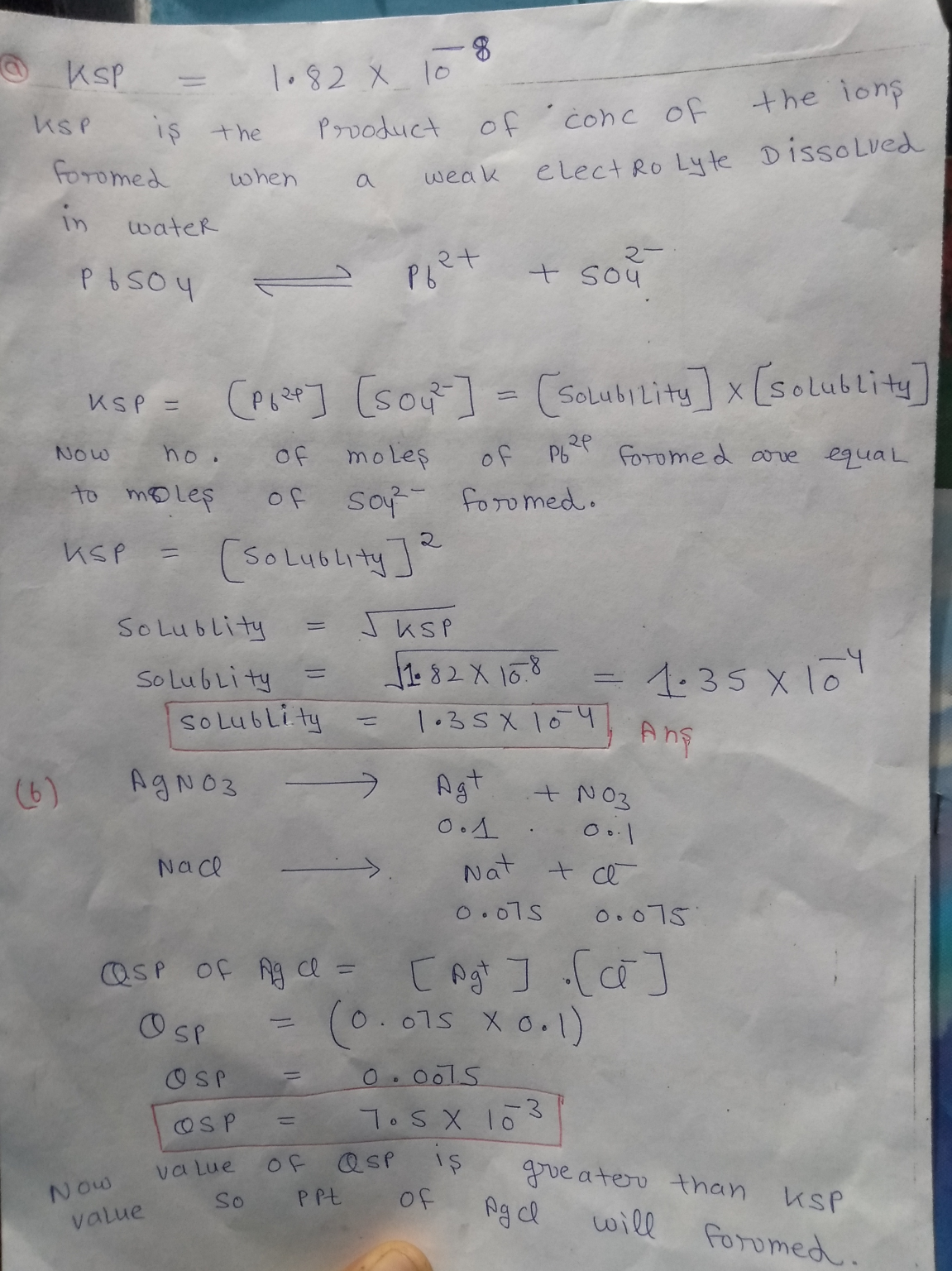#### Earn Coin

Coins can be redeemed for fabulous gifts.

Similar Homework Help Questions
• ### Determine the molar solubility of PbSO4 in pure water. Ksp(PbSO4)= 1.82 x 10^-8.

Determine the molar solubility of PbSO4 in pure water. Ksp(PbSO4)= 1.82 x 10^-8.

• ### Which of the following compounds will have the highest molar solubility in pure water? PbSO4, Ksp = 1.82

Which of the following compounds will have the highest molar solubility in pure water? PbSO4, Ksp = 1.82

• ### 8. (1pt) Determine the molar solubility of PbSO, in pure water. Ksp (PbSO4) = 1.82 * 10-8 a. 1.82 * 10M C. 9.1 x 10...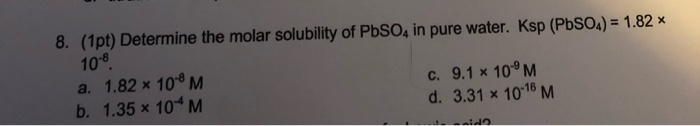8. (1pt) Determine the molar solubility of PbSO, in pure water. Ksp (PbSO4) = 1.82 * 10-8 a. 1.82 * 10M C. 9.1 x 10°M b. 1.35 * 10*M d. 3.31 x 10-18 M

• ### A solution of AgNO3 is mixed with NaCl to form a solution that is 0.10 M in AgNO3 and 0.075 M in NaCl. What will happen...

A solution of AgNO3 is mixed with NaCl to form a solution that is 0.10 M in AgNO3 and 0.075 M in NaCl. What will happen once these solutions are mixed? Ksp (AgCl) = 1.77

• ### Determine the molar solubility of PbSO4 in pure water. Ksp (PbSO4) = 1.6 × 10-10. a) 4.12 × 10-5 M b) 1.35 × 10-4 M c) 4...

Determine the molar solubility of PbSO4 in pure water. Ksp (PbSO4) = 1.6 × 10-10. a) 4.12 × 10-5 M b) 1.35 × 10-4 M c) 4.89 × 10-4 M d) 1.26 × 10-5 M e) 1.28 × 10-8 M

• ### Determine the molar solubility of AgBr in: Ksp (AgBr) = 7.7 x 10^ -13 a. a solution of pure water b. a solution conta...

Determine the molar solubility of AgBr in: Ksp (AgBr) = 7.7 x 10^ -13 a. a solution of pure water b. a solution containing 0.150 M NaBr c. A solution containing 0.25 M NaCL

• ### 14. What is the molar solubility of AgCl in pure water at 25°C? Ksp =1.8 x 10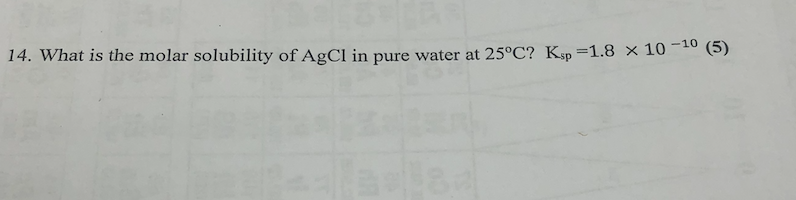14. What is the molar solubility of AgCl in pure water at 25°C? Ksp =1.8 x 10

• ### 1. Consider two nearly insoluble silver salts, silver chlor FINAL Equilibrium Practice Set molar solubility of...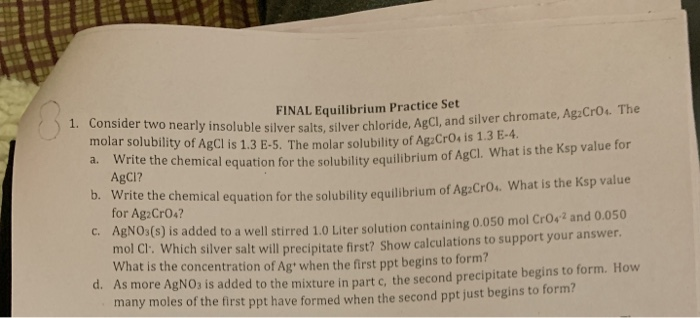1. Consider two nearly insoluble silver salts, silver chlor FINAL Equilibrium Practice Set molar solubility of AgCl is 1.3 E-5. The molar solubility of AgaC puble silver salts, silver chloride, AgCl, and silver chromate, AgzCros. The E-5. The molar solubility of AgaCrocis 1.3 E-4. a. Write the the chemical equation for the solubility equilibrium of AgCl. What is the Ksp value for AgCl? b. Write the chemical equation for the solubility equi chemical equation for the solubility equilibrium of AgaCros....

• ### The Ksp of PbBr2 is 6.60*10^-6 What is the molar solubility(M) of PbBr2 in pure water?...

The Ksp of PbBr2 is 6.60*10^-6 What is the molar solubility(M) of PbBr2 in pure water? What is the molar solubility(M) of PbBr2 in 0.500M KBr solution? What is the molar solubility(M) of PbBr2 in a 0.500M Pb(NO3)2 solution?

• ### The ksp of pbbr2 is 6.60 10 6. What is the molar solubility(M) of PbBr2 in pure water?

The Ksp of PbBr2 is 6.60*10^-6 What is the molar solubility(M) of PbBr2 in pure water? What is the molar solubility(M) of PbBr2 in 0.500M KBr solution? What is the molar solubility(M) of PbBr2 in a 0.500M Pb(NO3)2 solution?